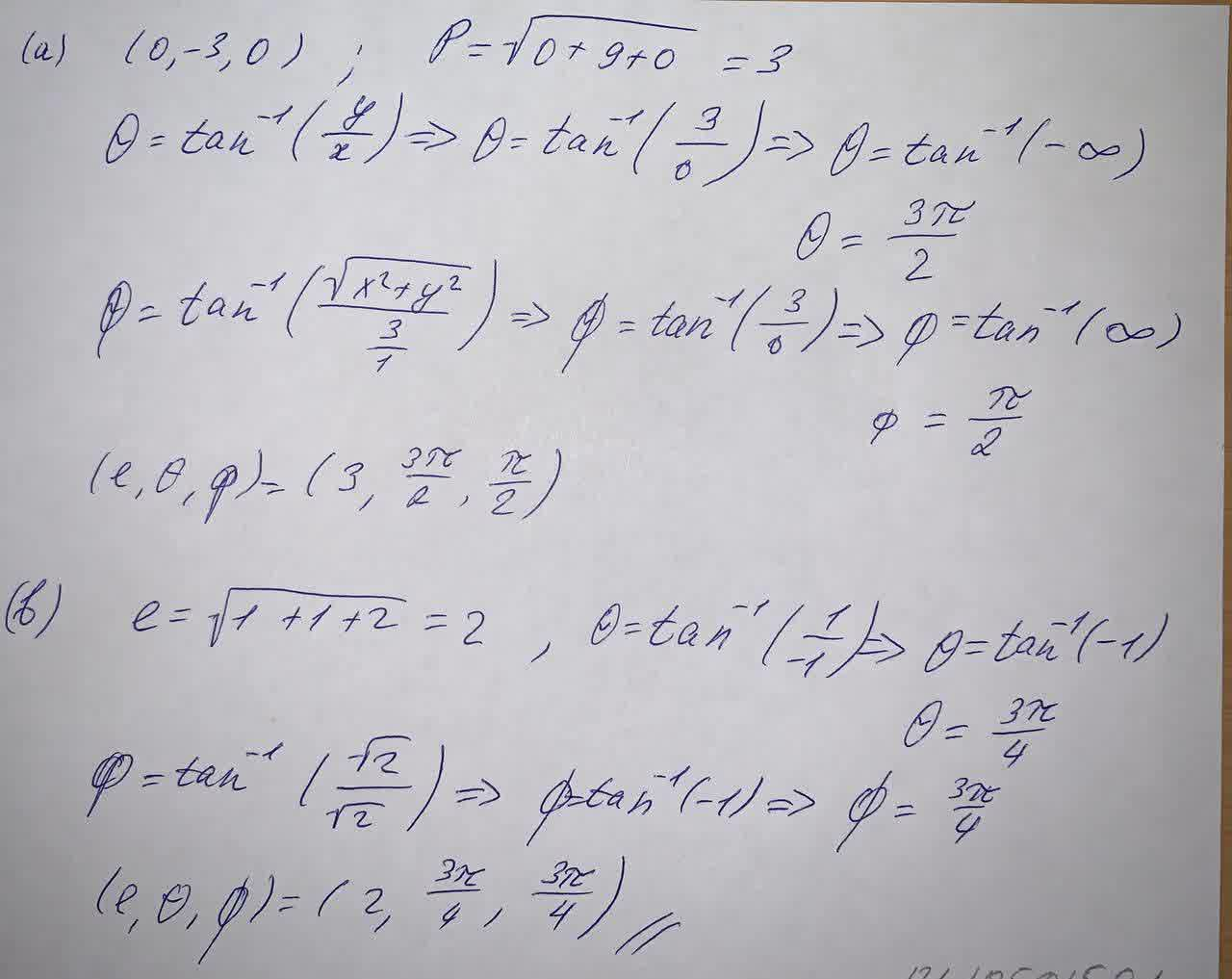Question# Change from rectangular to spherical coordinates. (Let \rho\geq 0, 0 \leq \theta \leq 2\pi, \text{ and } 0 \leq \phi \leq \pi)

Parametric equations
ANSWEREDChange from rectangular to spherical coordinates. (Let $$\displaystyle\rho\geq{0},{0}\leq\theta\leq{2}\pi,\ \text{ and }\ {0}\leq\phi\leq\pi$$)
(a) $$(0, -3, 0)$$
(b) $$\displaystyle{\left(-{1},{1},-\sqrt{{{2}}}\right)}$$